# A Bayesian Model of Lego Set Ratings

Over the last few months I have developed an interest in analyzing data about Lego sets, primarily scraped from the excellent community resource Brickset. Until now I have focused on price, with one empirical analysis and two Bayesian analyses of the factors that drive Lego set prices, fairness of the pricing of certain notable sets (75296 and 75309), and whether I tend to collect over- or under-priced sets.

In this post I will change that focus to understanding what factors affect the ratings given to each set by Brickset members. Given my particular interest in Star Wars sets (they comprise the overwhelming majority of my collection), I am specifically curious if there is any relationship between the critical and popular reception of individual Star Wars films and television series and the Lego sets associated with them.

First we make the necessary Python imports and do some light housekeeping.

In :
%matplotlib inline

In :
import datetime
from functools import reduce
from warnings import filterwarnings

In :
import arviz as az
from aesara import shared, tensor as at
import matplotlib as mpl
from matplotlib import pyplot as plt
from matplotlib.ticker import MultipleLocator, StrMethodFormatter
import networkx as nx
import numpy as np
import pandas as pd
import pymc as pm
import scipy as sp
import seaborn as sns
from sklearn.preprocessing import StandardScaler
import xarray as xr

You are running the v4 development version of PyMC which currently still lacks key features. You probably want to use the stable v3 instead which you can either install via conda or find on the v3 GitHub branch: https://github.com/pymc-devs/pymc/tree/v3

In :
filterwarnings('ignore', category=RuntimeWarning, module='aesara')
filterwarnings('ignore', category=UserWarning, module='arviz')
filterwarnings('ignore', category=FutureWarning, module='pymc')

In :
FIG_WIDTH = 8
FIG_HEIGHT = 6
mpl.rcParams['figure.figsize'] = (FIG_WIDTH, FIG_HEIGHT)

sns.set(color_codes=True)

pct_formatter = StrMethodFormatter('{x:.1%}')


We begin the real work by loading the data scraped from Brickset. See the first post in this series for more background on the data. Note that in addition to the data present in previous scrapes we have added the count of star ratings '✭', '✭✭','✭✭✭', '✭✭✭✭', and '✭✭✭✭✭' for each set that has ratings available.

In :
DATA_URI = 'https://austinrochford.com/resources/lego/brickset_19800101_20210922.csv.gz'

In :
def to_year(x):
return np.int64(round(x))

In :
ATTR_COLS = [
'Set number', 'Name', 'Set type', 'Year released',
'Theme', 'Subtheme', 'Pieces', 'RRP$' ] RATING_COLS = ['✭', '✭✭','✭✭✭', '✭✭✭✭', '✭✭✭✭✭'] ALL_COLS = ATTR_COLS + RATING_COLS  In : full_df = (pd.read_csv(DATA_URI, usecols=ALL_COLS) [ALL_COLS] .dropna(subset=set(ATTR_COLS) - {"Subtheme"})) full_df["Year released"] = full_df["Year released"].apply(to_year) full_df["Subtheme"] = full_df["Subtheme"].fillna("None") full_df = (full_df.sort_values(["Year released", "Set number"]) .set_index("Set number"))  We see that the data set contains information on approximately 8,600 Lego sets produced between 1980 and September 2021. In : full_df  Out: Name Set type Year released Theme Subtheme Pieces RRP$ ✭✭ ✭✭✭ ✭✭✭✭ ✭✭✭✭✭
Set number
1041-2 Educational Duplo Building Set Normal 1980 Dacta None 68.0 36.50 NaN NaN NaN NaN NaN
1075-1 LEGO People Supplementary Set Normal 1980 Dacta None 304.0 14.50 NaN NaN NaN NaN NaN
1101-1 Replacement 4.5V Motor Normal 1980 Service Packs None 1.0 5.65 NaN NaN NaN NaN NaN
1123-1 Ball and Socket Couplings & One Articulated Joint Normal 1980 Service Packs None 8.0 16.00 NaN NaN NaN NaN NaN
1130-1 Plastic Folder for Building Instructions Normal 1980 Service Packs None 1.0 14.00 NaN NaN NaN NaN NaN
... ... ... ... ... ... ... ... ... ... ... ... ...
80025-1 Sandy's Power Loader Mech Normal 2021 Monkie Kid Season 2 520.0 54.99 NaN NaN NaN NaN NaN
80026-1 Pigsy's Noodle Tank Normal 2021 Monkie Kid Season 2 662.0 59.99 NaN NaN NaN NaN NaN
80028-1 The Bone Demon Normal 2021 Monkie Kid Season 2 1375.0 119.99 NaN NaN NaN NaN NaN
80106-1 Story of Nian Normal 2021 Seasonal Chinese Traditional Festivals 1067.0 79.99 NaN 2.0 2.0 11.0 14.0
80107-1 Spring Lantern Festival Normal 2021 Seasonal Chinese Traditional Festivals 1793.0 119.99 1.0 1.0 2.0 14.0 82.0

8632 rows × 12 columns

In :
full_df.describe()

Out:
Year released Pieces RRP$✭✭ ✭✭✭ ✭✭✭✭ ✭✭✭✭✭ count 8632.000000 8632.000000 8632.000000 5025.000000 5476.000000 5667.000000 5694.000000 5693.000000 mean 2009.286376 263.574490 31.667443 4.649154 8.555698 19.518087 30.423077 45.170912 std 9.030748 489.757624 45.678743 4.126446 7.975121 18.917916 32.934498 62.309390 min 1980.000000 0.000000 0.000000 1.000000 1.000000 1.000000 1.000000 1.000000 25% 2003.000000 32.000000 7.000000 2.000000 3.000000 7.000000 11.000000 13.000000 50% 2012.000000 100.000000 18.000000 3.000000 6.000000 14.000000 20.000000 27.000000 75% 2017.000000 305.000000 39.990000 6.000000 11.000000 26.000000 37.750000 52.000000 max 2021.000000 11695.000000 799.990000 46.000000 96.000000 228.000000 361.000000 1080.000000 As in previous posts, we adjust RRP (recommended retail price) to 2021 dollars. In : CPI_URL = 'https://austinrochford.com/resources/lego/CPIAUCNS202100401.csv'  In : years = pd.date_range('1979-01-01', '2021-01-01', freq='Y') \ + datetime.timedelta(days=1) cpi_df = (pd.read_csv(CPI_URL, index_col="DATE", parse_dates=["DATE"]) .loc[years]) cpi_df["to2021"] = cpi_df.loc["2021-01-01"] / cpi_df cpi_df["year"] = cpi_df.index.year  In : cpi_df.head()  Out: CPIAUCNS to2021 year DATE 1980-01-01 77.8 3.362237 1980 1981-01-01 87.0 3.006690 1981 1982-01-01 94.3 2.773934 1982 1983-01-01 97.8 2.674663 1983 1984-01-01 101.9 2.567046 1984 In : pd.merge(full_df, cpi_df, left_on="Year released", right_on="year")  Out: Name Set type Year released Theme Subtheme Pieces RRP$ ✭✭ ✭✭✭ ✭✭✭✭ ✭✭✭✭✭ CPIAUCNS to2021 year
0 Educational Duplo Building Set Normal 1980 Dacta None 68.0 36.50 NaN NaN NaN NaN NaN 77.800 3.362237 1980
1 LEGO People Supplementary Set Normal 1980 Dacta None 304.0 14.50 NaN NaN NaN NaN NaN 77.800 3.362237 1980
2 Replacement 4.5V Motor Normal 1980 Service Packs None 1.0 5.65 NaN NaN NaN NaN NaN 77.800 3.362237 1980
3 Ball and Socket Couplings & One Articulated Joint Normal 1980 Service Packs None 8.0 16.00 NaN NaN NaN NaN NaN 77.800 3.362237 1980
4 Plastic Folder for Building Instructions Normal 1980 Service Packs None 1.0 14.00 NaN NaN NaN NaN NaN 77.800 3.362237 1980
... ... ... ... ... ... ... ... ... ... ... ... ... ... ... ...
8627 Sandy's Power Loader Mech Normal 2021 Monkie Kid Season 2 520.0 54.99 NaN NaN NaN NaN NaN 261.582 1.000000 2021
8628 Pigsy's Noodle Tank Normal 2021 Monkie Kid Season 2 662.0 59.99 NaN NaN NaN NaN NaN 261.582 1.000000 2021
8629 The Bone Demon Normal 2021 Monkie Kid Season 2 1375.0 119.99 NaN NaN NaN NaN NaN 261.582 1.000000 2021
8630 Story of Nian Normal 2021 Seasonal Chinese Traditional Festivals 1067.0 79.99 NaN 2.0 2.0 11.0 14.0 261.582 1.000000 2021
8631 Spring Lantern Festival Normal 2021 Seasonal Chinese Traditional Festivals 1793.0 119.99 1.0 1.0 2.0 14.0 82.0 261.582 1.000000 2021

8632 rows × 15 columns

In :
full_df.insert(len(ATTR_COLS) - 1,
"RRP2021",
(pd.merge(full_df, cpi_df,
left_on="Year released",
right_on="year")
.apply(lambda df: (df["RRP$"] * df["to2021"]), axis=1)) .values)  In : full_df.head()  Out: Name Set type Year released Theme Subtheme Pieces RRP$ RRP2021 ✭✭ ✭✭✭ ✭✭✭✭ ✭✭✭✭✭
Set number
1041-2 Educational Duplo Building Set Normal 1980 Dacta None 68.0 36.50 122.721632 NaN NaN NaN NaN NaN
1075-1 LEGO People Supplementary Set Normal 1980 Dacta None 304.0 14.50 48.752429 NaN NaN NaN NaN NaN
1101-1 Replacement 4.5V Motor Normal 1980 Service Packs None 1.0 5.65 18.996636 NaN NaN NaN NaN NaN
1123-1 Ball and Socket Couplings & One Articulated Joint Normal 1980 Service Packs None 8.0 16.00 53.795784 NaN NaN NaN NaN NaN
1130-1 Plastic Folder for Building Instructions Normal 1980 Service Packs None 1.0 14.00 47.071311 NaN NaN NaN NaN NaN

We also add a column indicating whether or not I own each set.

In :
AUSTIN_URI = 'https://austinrochford.com/resources/lego/Brickset-MySets-owned-20211002.csv'

In :
austin_sets = set(
.values
.squeeze()
)

In :
full_df["Austin owns"] = (full_df.index
.get_level_values("Set number")
.isin(austin_sets))

In :
full_df.head()

Out:
Name Set type Year released Theme Subtheme Pieces RRP$RRP2021 ✭✭ ✭✭✭ ✭✭✭✭ ✭✭✭✭✭ Austin owns Set number 1041-2 Educational Duplo Building Set Normal 1980 Dacta None 68.0 36.50 122.721632 NaN NaN NaN NaN NaN False 1075-1 LEGO People Supplementary Set Normal 1980 Dacta None 304.0 14.50 48.752429 NaN NaN NaN NaN NaN False 1101-1 Replacement 4.5V Motor Normal 1980 Service Packs None 1.0 5.65 18.996636 NaN NaN NaN NaN NaN False 1123-1 Ball and Socket Couplings & One Articulated Joint Normal 1980 Service Packs None 8.0 16.00 53.795784 NaN NaN NaN NaN NaN False 1130-1 Plastic Folder for Building Instructions Normal 1980 Service Packs None 1.0 14.00 47.071311 NaN NaN NaN NaN NaN False Based on the exploratory data analysis in the first post in this series, we filter full_df down to approximately 6,400 sets to be considered for analysis. In : FILTERS = [ full_df["Set type"] == "Normal", full_df["Pieces"] > 10, full_df["Theme"] != "Duplo", full_df["Theme"] != "Service Packs", full_df["Theme"] != "Bulk Bricks", full_df["RRP2021"] > 0 ]  In : df = full_df[reduce(np.logical_and, FILTERS)].copy()  In : df  Out: Name Set type Year released Theme Subtheme Pieces RRP$ RRP2021 ✭✭ ✭✭✭ ✭✭✭✭ ✭✭✭✭✭ Austin owns
Set number
1041-2 Educational Duplo Building Set Normal 1980 Dacta None 68.0 36.50 122.721632 NaN NaN NaN NaN NaN False
1075-1 LEGO People Supplementary Set Normal 1980 Dacta None 304.0 14.50 48.752429 NaN NaN NaN NaN NaN False
5233-1 Bedroom Normal 1980 Homemaker None 26.0 4.50 15.130064 NaN NaN NaN NaN NaN False
6305-1 Trees and Flowers Normal 1980 Town Accessories 12.0 3.75 12.608387 3.0 4.0 9.0 6.0 13.0 False
6306-1 Road Signs Normal 1980 Town Accessories 12.0 2.50 8.405591 4.0 2.0 8.0 13.0 17.0 False
... ... ... ... ... ... ... ... ... ... ... ... ... ... ...
80025-1 Sandy's Power Loader Mech Normal 2021 Monkie Kid Season 2 520.0 54.99 54.990000 NaN NaN NaN NaN NaN False
80026-1 Pigsy's Noodle Tank Normal 2021 Monkie Kid Season 2 662.0 59.99 59.990000 NaN NaN NaN NaN NaN False
80028-1 The Bone Demon Normal 2021 Monkie Kid Season 2 1375.0 119.99 119.990000 NaN NaN NaN NaN NaN False
80106-1 Story of Nian Normal 2021 Seasonal Chinese Traditional Festivals 1067.0 79.99 79.990000 NaN 2.0 2.0 11.0 14.0 False
80107-1 Spring Lantern Festival Normal 2021 Seasonal Chinese Traditional Festivals 1793.0 119.99 119.990000 1.0 1.0 2.0 14.0 82.0 False

6420 rows × 14 columns

In :
df.describe()

Out:
Year released Pieces RRP$RRP2021 ✭✭ ✭✭✭ ✭✭✭✭ ✭✭✭✭✭ count 6420.000000 6420.000000 6420.000000 6420.000000 4307.000000 4712.000000 4883.000000 4907.000000 4906.000000 mean 2009.714642 343.184112 37.552062 46.171183 4.636638 8.516978 19.449928 31.193397 46.018956 std 8.939369 544.179590 50.379534 59.373029 4.273900 8.313056 19.843605 34.925100 66.244232 min 1980.000000 11.000000 0.600000 0.971220 1.000000 1.000000 1.000000 1.000000 1.000000 25% 2003.000000 69.000000 9.990000 11.870620 2.000000 3.000000 7.000000 10.000000 13.000000 50% 2012.000000 181.000000 19.990000 27.347055 3.000000 6.000000 13.000000 19.000000 25.000000 75% 2017.000000 404.000000 49.990000 56.497192 6.000000 11.000000 25.000000 38.000000 52.000000 max 2021.000000 11695.000000 799.990000 897.373477 46.000000 96.000000 228.000000 361.000000 1080.000000 We now reduce df to sets that have had at least one rating. In : rated_df = df.dropna(how='all', subset=RATING_COLS).copy() rated_df[RATING_COLS] = rated_df[RATING_COLS].fillna(0.) rated_df["Ratings"] = rated_df[RATING_COLS].sum(axis=1)  In : rated_df.head()  Out: Name Set type Year released Theme Subtheme Pieces RRP$ RRP2021 ✭✭ ✭✭✭ ✭✭✭✭ ✭✭✭✭✭ Austin owns Ratings
Set number
6305-1 Trees and Flowers Normal 1980 Town Accessories 12.0 3.75 12.608387 3.0 4.0 9.0 6.0 13.0 False 35.0
6306-1 Road Signs Normal 1980 Town Accessories 12.0 2.50 8.405591 4.0 2.0 8.0 13.0 17.0 False 44.0
6375-2 Exxon Gas Station Normal 1980 Town Shops and Services 267.0 20.00 67.244730 0.0 0.0 1.0 5.0 12.0 False 18.0
6390-1 Main Street Normal 1980 Town Shops and Services 591.0 40.00 134.489460 0.0 1.0 1.0 3.0 14.0 False 19.0
6861-1 X1 Patrol Craft Normal 1980 Space Classic 55.0 4.00 13.448946 1.0 3.0 6.0 14.0 19.0 False 43.0

We see that slightly more than 75% of the sets under consideration have at least one rating, which is (pleasantly) more than I was expecting.

In :
rated_df.shape / df.shape

Out:
0.7643302180685358

### Exploratory Data Analysis¶

We begin with a descriptive exploration of the Brickset ratings data. Immediately we see that the vast majority of sets have been rated a few dozen to a few hundred times.

In :
fig, axes = plt.subplots(ncols=2, sharex=True,
figsize=(1.75 * FIG_WIDTH, FIG_HEIGHT))

sns.histplot(rated_df[RATING_COLS].sum(axis=1),
lw=0., ax=axes)

axes.set_xlim(left=0);
axes.set_xlabel("Number of ratings");

axes.set_ylabel("Number of sets");

sns.histplot(rated_df[RATING_COLS].sum(axis=1),
cumulative=True, lw=0., ax=axes)
axes.axhline(rated_df.shape, c='k', ls='--');

axes.set_xlim(left=0);
axes.set_xlabel("Number of ratings");

axes.set_ylabel("Cumulative number of sets");

fig.tight_layout();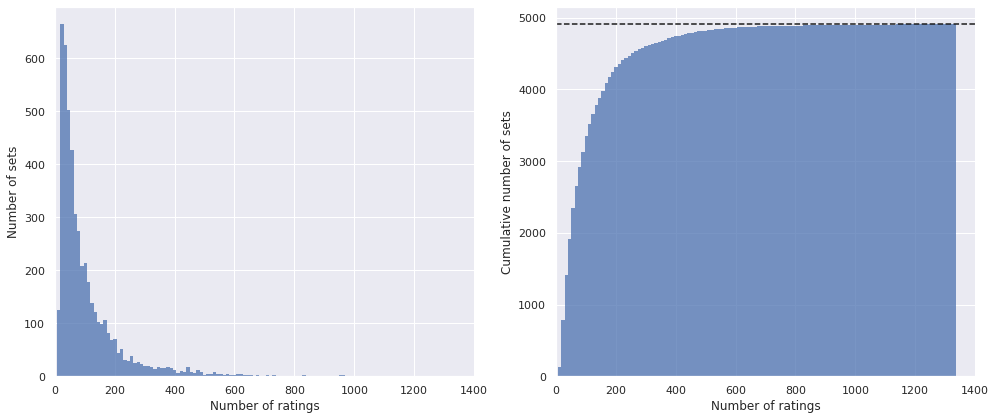We now explore the distribution of average ratings.

In :
def average_rating(ratings):
if isinstance(ratings, (xr.DataArray, xr.Dataset)):
stars = ratings.coords["rating"].str.len()

return (ratings * stars).sum(dim="rating") / ratings.sum(dim="rating")
else:
ratings = np.asanyarray(ratings)
stars = 1 + np.arange(ratings.shape[-1])

return (ratings * stars).sum(axis=-1) / ratings.sum(axis=-1)

In :
rated_df["Average rating"] = (rated_df[RATING_COLS]
.apply(average_rating, axis=1))


We see that the distribution over time (our data set covers sets released between 1980 and September 2021) has decreased from just under 4.5 to just below 4 over the years. Unsurprisingly, the percentage of ratings in each category between one and five stars has varied similarly over time.

In :
time_fig, axes = plt.subplots(
ncols=2, sharex=True,
figsize=(1.75 * FIG_WIDTH, FIG_HEIGHT)
)

(rated_df["Average rating"]
.groupby(rated_df["Year released"])
.mean()
.rolling(5, min_periods=1)
.mean()
.plot(ax=axes));

axes.set_ylim(3.4, 4.6);
axes.set_yticks([3.5, 4, 4.5]);
axes.set_yticklabels(["✭✭✭ ½", "✭✭✭✭", "✭✭✭✭ ½"]);
axes.set_ylabel("Average set rating");

(rated_df[RATING_COLS]
.div(rated_df[RATING_COLS].sum(axis=1),
axis=0)
.groupby(rated_df["Year released"])
.mean()
.rolling(5, min_periods=1)
.mean()
.iloc[:, ::-1]
.plot(ax=axes));

axes.yaxis.set_major_formatter(pct_formatter);
axes.set_ylabel("Average percentage of ratings");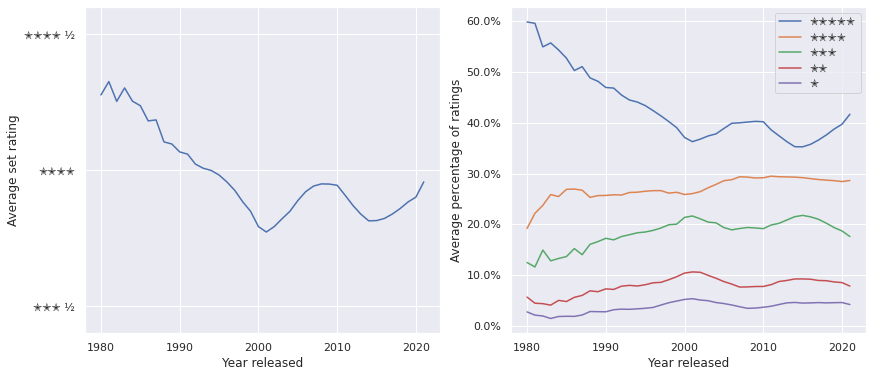It is interesting to try to interpret this trend. Since the year is the year the set was released and not necessarily reviewed (Brickset did not exist until 1997), there is certainly some selection bias in early reviews, because they will only exist for those Lego collectors sufficiently passionate to own older sets, join Brickset, and rate them there once the set becomes available. In fact, for any period of time it is important to remember that these ratings do not represent the sentiment of the general Lego purchasing or collecting public towards each set, but the sentiments of the subset of those purchasers and collectors that are not only movitated to visit and join Brickset, but to rate their sets there. For reference, I visit Bricket frequently for both research purposes and to track my collection, but I have never rated a set there. It seems reasonable to assume that these biases will shrink, but never truly vanish, for more recently released sets. These biases and caveats are important to keep in the back of our mind as we perform our analysis.

We now turn to the specific (sub)themes that I tend to collect, namely Star Wars and NASA sets.

In :
fig, axes = plt.subplots(ncols=2, sharex=True, sharey=True,
figsize=(1.75 * FIG_WIDTH, FIG_HEIGHT))

# Theme
sns.kdeplot(
rated_df.groupby("Theme")
["Average rating"]
.mean(),
ax=axes
);
sns.rugplot(
rated_df.groupby("Theme")
["Average rating"]
.mean(),
height=0.05, c='C0', ax=axes
);
axes.axvline(
rated_df["Average rating"]
.mean(),
ls='--', label="Average (all themes)"
);
axes.axvline(
rated_df[rated_df["Theme"] == "Star Wars"]
["Average rating"]
.mean(),
color='C1', label="Star Wars sets"
);

axes.set_yticks([]);
axes.legend(loc='upper left');
axes.set_title("Average rating by theme");

# Subtheme
sns.kdeplot(
rated_df.groupby("Subtheme")
["Average rating"]
.mean(),
ax=axes
);
sns.rugplot(
rated_df.groupby("Subtheme")
["Average rating"]
.mean(),
height=0.05, c='C0', ax=axes
);
axes.axvline(
rated_df["Average rating"]
.mean(),
ls='--', label="Average (all subthemes)"
);
axes.axvline(
rated_df[rated_df["Subtheme"] == "NASA"]
["Average rating"]
.mean(),
color='C2', label="NASA sets"
);

axes.legend(loc='upper left');
axes.set_title("Average rating by theme");

fig.tight_layout();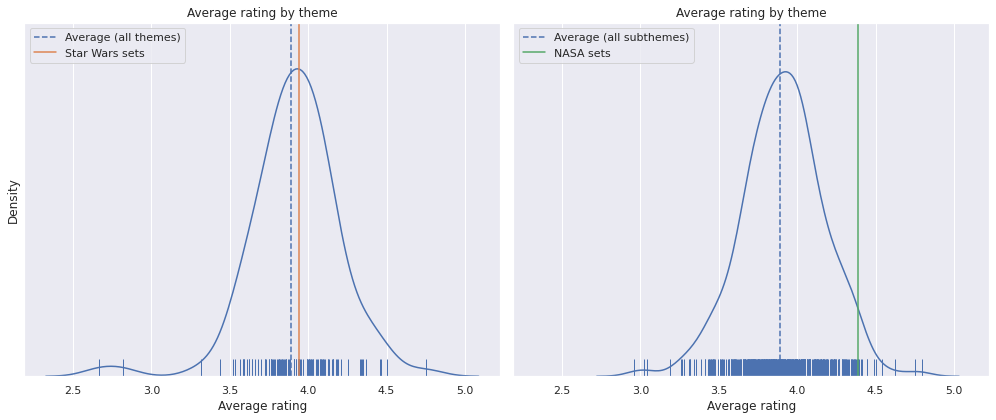We see that the average rating for Star Wars sets is close to the average rating of all sets, whereas the averating rating for NASA sets is significantly higher than the average rating for all sets. I am not surprised that NASA sets score so highly (21309, 10266, 10283, and 21312) are some of my favorite sets, but I am a bit surprised to find that Star Wars, as an overall theme, is as middle-of-the-road as it is. Even restricting to the period after 1999 (when Star Wars sets started to be released), this phenomenon persists.

In :
star_wars_min_year = (rated_df[rated_df["Theme"] == "Star Wars"]
["Year released"]
.min())

In :
star_wars_min_year

Out:
1999
In :
ax = sns.kdeplot(
rated_df.groupby("Theme")
["Average rating"]
.mean(),
)
sns.rugplot(
rated_df[rated_df["Year released"] >= star_wars_min_year]
.groupby("Theme")
["Average rating"]
.mean(),
height=0.05, c='C0', ax=ax
);
ax.axvline(
rated_df[rated_df["Year released"] >= star_wars_min_year]
["Average rating"]
.mean(),
ls='--', label="Average (all themes)"
);
ax.axvline(
rated_df[rated_df["Theme"] == "Star Wars"]
["Average rating"]
.mean(),
color='C1', label="Star Wars sets"
);

ax.set_yticks([]);
ax.legend(loc='upper left');
ax.set_title(f"Average rating by theme\n(Sets released after {star_wars_min_year})");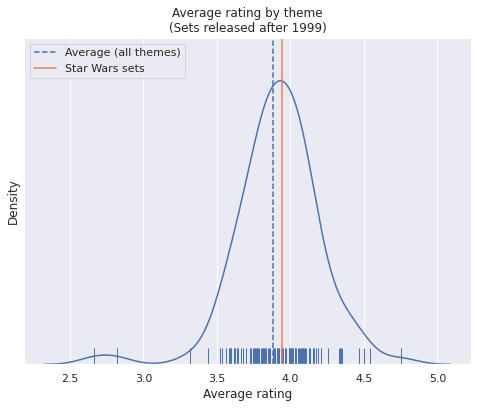Our subsequent analysis will attempt to control for both the year a set was released and other factors (piece count and price) more rigorously in order to see if this phenomenon persists.

Turning to piece count and price, we see that a positive, fairly linear relationship between both of these characteristics and average rating.

In :
pieces_price_grid = sns.pairplot(rated_df,
x_vars=["Pieces", "RRP2021"],
y_vars=["Average rating"],
plot_kws={'alpha': 0.25},
height=FIG_HEIGHT)

for ax in pieces_price_grid.axes.flat:
ax.set_xscale('log');

pieces_price_grid.axes[0, 1].set_xlabel("RRP (2021 $)");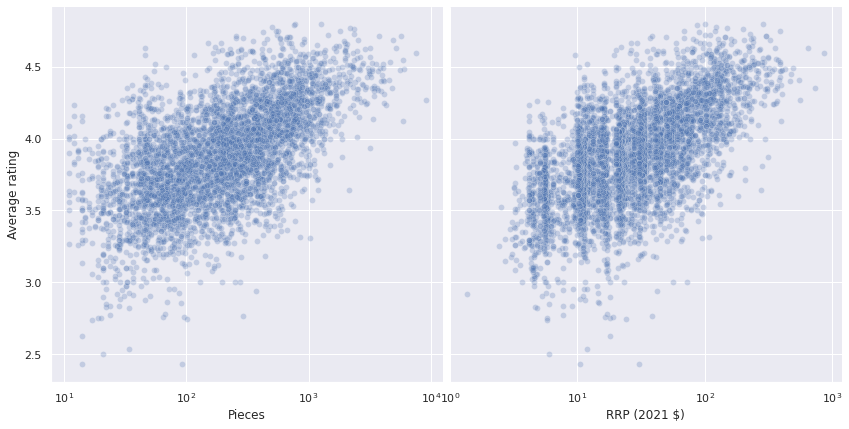It is interesting to consider the causal nature of the relationship between piece count, price, and ratings. Clearly larger sets requiring more pieces have higher production costs and therefore higher retail prices. Higher priced sets probably also have to be of a better overall quality in order to justify the significant expense. I personally occasionally buy cheaper sets that I don't love if they have interesting components. I purchased 75299 just to get Mando and the Child riding a speeder together (the rest of the bags went straight into my spare parts bin).Based on this discussion, the causal DAG for the relationship between piece count, price, and rating is as follows. In : graph = nx.DiGraph() graph.add_edges_from([ ("Piece count", "Price"), ("Piece count", "Rating"), ("Price", "Rating") ])  In : fig, ax = plt.subplots() POS = { "Piece count": (1, 1), "Price": (0, 0.5), "Rating": (1, 0) } LABEL_POS = { "Piece count": (0.97, 1.075), "Price": (0, 0.575), "Rating": (1, -0.075) } nx.draw_networkx_nodes(graph, pos=POS, ax=ax); nx.draw_networkx_edges(graph, pos=POS, ax=ax); nx.draw_networkx_labels(graph, pos=LABEL_POS, ax=ax); ax.set_xticks([]); ax.set_yticks([]); ax.set_aspect('equal');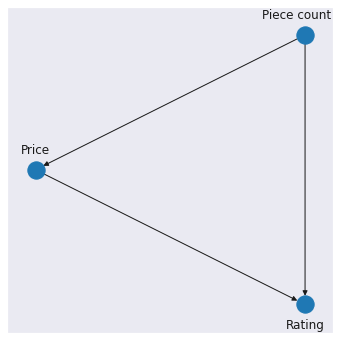This discussion of causality is certainly interesting and may be worth future exploration, but we will not take the causal perspective for the rest of this post. Rather, our models will be interpreted descriptively, as tools that help us summarize the ratings of various groups of Lego sets. We conclude our exploratory data analysis by seeing where the ratings of the sets I own fall in the distribution of all rated sets. It appears that most of my sets are rated above average, because, of course, I have excellent taste. In : ax = sns.kdeplot(rated_df["Average rating"]) sns.rugplot(rated_df["Average rating"], height=0.05, c='C0', ax=ax); ax.axvline(rated_df["Average rating"].mean(), c='C0', ls='--', label="Average (all sets)"); sns.rugplot( rated_df[rated_df["Austin owns"]] ["Average rating"], height=0.05, c='C1', ax=ax ); ax.axvline( rated_df[rated_df["Austin owns"]] ["Average rating"] .mean(), c='C1', label="Average (my sets)" ); ax.set_yticks([]); ax.legend();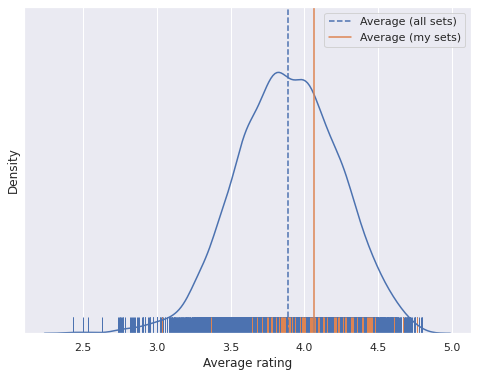### Modeling Ratings¶ We will now build a few Bayesian models gradually incorporating the relationships we found during exploratory data analysis. The appropriate model for this type of data (ratings on a one-to-five star scale) is an ordinal regression model. While the ratings are ostensibly numerical, and we may be tempted to model them using, for example, a normal likelihood, an ordinal regression model is more appropriate. While it is fairly safe to assume that a set I like a set that I give four stars better than a set that I give five starts, it is a much bigger assumption to say that the difference in how much I like these two sets is exactly the same as the difference between two sets that I rate with four and five stars just because$4 - 3 = 5 - 4$. Ordinal regression is a flexible model that acknowledges that the responses are ordered, but allows the distance between the categories of response to vary. Mathematically an ordinal regression model can be specified as followed. If we have$K$response classes (in our case$K = 5$), we define$K - 1$ascending cut points$c_1 < c_2 < \ldots < c_{K - 1}$. Let$g: (0, 1) \to \mathbb{R}$be a link function with$\displaystyle{\lim_{\eta \to 0}}\ g(\eta) = -\infty$and$\displaystyle{\lim_{\eta \to 1}}\ g(\eta) = \infty$. The logit and probit link functions are common choices, leading to ordinal regression models with slightly different interpretations. If$\eta_i$is a latent quantity related to the$i$-th observed rating (specifying the form of$\eta_i$is the most important part of our model and will occupy much of the rest of this post), then the probability that the rating$R_i$is at most$k \in {1, 2, \ldots K}$is $$P(R_i \leq k\ |\ \eta_i) = g^{-1}(c_k - \eta_i).$$ If we define$c_0 = -\infty$,$c_K = \infty$,$g^{-1}(-\infty) = 0$and$g^{-1}(\infty) = 1$, we get $$P(R_i = k\ |\ \eta_i) = P(R_i \leq k\ |\ \eta_i) - P(R_i \leq k - 1\ |\ \eta_i) = g^{-1}(c_k - \eta_i) - g^{-1}(c_{k - 1} - \eta_i)$$ . To concretely illustrate the ordinal regression model, suppose there are$K = 3$possible ratings,$c_1 = 0$,$c_2 = 2.3$, and we use the logistic link $$g^{-1}(\eta) = \frac{1}{1 + \exp(-\eta)}.$$ An example of the rating probabilities given$\eta$are shown below. In : C = np.array([0., 2.3])  In : η_plot = np.linspace(-2, 4, 100) η_diff = (-np.subtract.outer(η_plot, C)) p_plot = np.diff(sp.special.expit(η_diff), prepend=0., append=1.)  In : fig, ax = plt.subplots() for k, c_plot in enumerate(C, start=1): ax.axvline(c_plot, c='k', ls='--', zorder=5, label=f"$c_{{{k}}} = {c_plot}$"); for k, p_plot_ in enumerate(p_plot.T): ax.plot(η_plot, p_plot_, label=f"$k = {k}$"); ax.set_xlim(-2, 4); ax.set_xlabel(r"$\eta$"); ax.set_ylim(0, 1); ax.yaxis.set_major_formatter(pct_formatter); ax.set_ylabel(r"$P(R = k \|\ \eta)$"); ax.legend(); ax.set_title("Ordinal Regression Probabilities");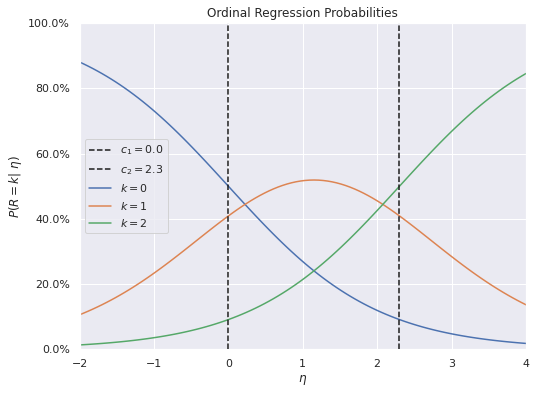Those familiar with common applications of ordinal regression and/or psychometrics will recognize that we are quite close to having specified an ordered item-response model. In a typical ordinal item-response model, the cut points$c_k$are allowed to vary according to the item being rated and the latent quantity$\eta$is allowed to vary according to the item being rated and the person doing the rating. Unfortunately with our Brickset data, we have no information about the individual raters so we cannot form an item-response model. Instead, we will infer a fixed set of cutpoints and allow$\eta$to vary based on the set being rated. Intriguingly, Brickset also allows members to leave reviews of specific sets which contain ratings along several dimensions. I have in fact scraped this review data, but exploratory data analysis shows that very few members rate more than one set, so I am not yet confident that I can build a useful item-response model using this data. Building such a model may be the topic of a future post, but for the moment we restrict our attention to the anonymous ratings we have explored above., #### Time¶ Our first model attempts to capture the temporal dynamics of ratings based on the year in which the set was released, as shown above. In : time_fig  Out:This focus on time (and set) effects will allow us to start by building a somewhat simple ordinal regression model in pymc3. This model will use smoothing splines to model the effect of year on ratings and set-level random effects to capture the popularity of a set relative to the baeline popularity of all sets in the year it was released. We now establish some notation. Throughout,$i$will denote the index of a set in rated_df. Let$N_i$be the number of times that set was rated and$\mathbf{R}_i = (R_{i, 1}, R_{i, 2}, R_{i, 3}, R_{i, 4}, R_{i, 5})$be the number of one-, two-, three-, four-, and five-star ratings for the$i$-th set, respectively. In : n_rating = len(RATING_COLS) n_cut = n_rating - 1 ratings_ct = rated_df[RATING_COLS].values ratings = rated_df["Ratings"].values  Let$t(i)$be (the index of) the year that the$i$-th set was released. In : t, year_map = rated_df["Year released"].factorize(sort=True) n_year = year_map.size  In our first model, we have $$\eta_i = f_{t(i)} + \beta_{\text{set}, i}.$$ Here$f_t$is a smoothing spline meant to capture the average rating of sets released in the$t$-th year. This post will not cover in depth the process for specifying a Bayesian smoothing spline. Interested readers should consult a previous post of mine for details. In : N_KNOT = 10 knots = np.linspace(0, n_year, N_KNOT) bf = sp.interpolate.BSpline(knots, np.eye(N_KNOT), 3) t_dmat = bf(np.arange(n_year))  In : coords = { "cut": np.arange(n_cut), "rating": RATING_COLS, "knot": np.arange(N_KNOT), "set": rated_df.index.values, "year": year_map }  In : SEED = 123456789 # for reproducibility  In : with pm.Model(coords=coords, rng_seeder=SEED) as model: β_t_inc = pm.Normal("β_t_inc", 0., 0.1, dims="knot") β_t = β_t_inc.cumsum() f_t = pm.Deterministic("f_t", at.dot(t_dmat, β_t), dims="year")  The set-level random effects$\beta_{\text{set}, i}follow a hierarchical normal distribution equivalent to \begin{align*} \sigma_{\beta_{\text{set}}} & \sim \text{Half}-N\left(2.5^2\right) \\ \beta_{\text{set}, i} & \sim N\left(0, \sigma_{\beta_{\text{set}}}^2\right). \end{align*} In practice, we use an equivalent non-centered parametrization that samples better in practice than this more mathematically elegant one. Note that it is important that the prior expected value of\eta_i$be fixed (in our case it is zero), otherwise model will not be identified. If the prior expected value is not fixed, adding any constant to the cutpoints and$\eta$will produce the same likelihood. In : # the scale necessary to make a halfnormal distribution # have unit variance HALFNORMAL_SCALE = 1. / np.sqrt(1. - 2. / np.pi) def noncentered_normal(name, *, dims, μ=None, σ=2.5): if μ is None: μ = pm.Normal(f"μ_{name}", 0., 2.5) Δ = pm.Normal(f"Δ_{name}", 0., 1., dims=dims) σ = pm.HalfNormal(f"σ_{name}", σ * HALFNORMAL_SCALE) return pm.Deterministic(name, μ + Δ * σ, dims=dims)  In : with model: β_set = noncentered_normal("β_set", dims="set", μ=0.) η = f_t[t] + β_set  We place a$N\left(0, 2.5^2\right)\$ squared prior on the cutpoints, constraining them to be ordered with the keyword argument transform=pm.transforms.ordered.

In :
with model:
c = pm.Normal("c", 0., 2.5, dims="cut",
transform=pm.transforms.ordered,
initval=coords["cut"])


It now remains to specify the likelihood of the observed rankings. Since the data we have is the count of ratings for each number of stars, an ordered multinomial model is appropriate.

In :
with model:
ratings_obs = pm.OrderedMultinomial(
"ratings_obs", η, c, ratings,
dims=("set", "rating"),
observed=ratings_ct
)


We are now ready to sample from this model's posterior distribution.

In :
CORES = 3

SAMPLE_KWARGS = {
'cores': CORES,
'random_seed': [SEED + i for i in range(CORES)]
}

In :
with model:
trace = pm.sample(**SAMPLE_KWARGS)

Auto-assigning NUTS sampler...
Multiprocess sampling (3 chains in 3 jobs)
NUTS: [β_t_inc, Δ_β_set, σ_β_set, c]

100.00% [6000/6000 20:39<00:00 Sampling 3 chains, 0 divergences]
Sampling 3 chains for 1_000 tune and 1_000 draw iterations (3_000 + 3_000 draws total) took 1242 seconds.
The number of effective samples is smaller than 10% for some parameters.


Standard sampling diagnostics show no cause for concern.

In :
az.plot_energy(trace);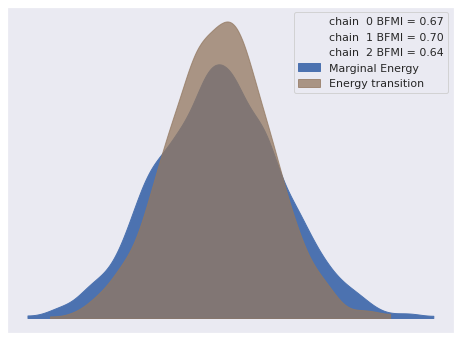In :
az.rhat(trace).max()

Out:
<xarray.Dataset>
Dimensions:            ()
Data variables:
β_t_inc            float64 1.026
Δ_β_set            float64 1.008
σ_β_set            float64 1.012
c                  float64 1.002
f_t                float64 1.005
β_set              float64 1.008
ratings_obs_probs  float64 1.008

The following plots show that this model has captured the temporal dynamics of set ratings fairly well.

In :
set_xr = (rated_df[["Year released", "Theme", "Subtheme"]]
.rename_axis("set")
.rename(columns={
"Year released": "year",
"Theme": "theme",
"Subtheme": "sub"
})
.to_xarray())

In :
fig, axes = plt.subplots(
ncols=2, sharex=True,
figsize=(1.75 * FIG_WIDTH, FIG_HEIGHT)
)

(rated_df["Average rating"]
.groupby(rated_df["Year released"])
.mean()
.rolling(5, min_periods=1)
.mean()
.plot(ax=axes));
(trace.posterior["ratings_obs_probs"]
.pipe(average_rating)
.mean(dim=("chain", "draw"))
.groupby(set_xr["year"])
.mean()
.to_dataframe(name="Posterior\nexpected value")
.rolling(5, min_periods=1)
.mean()
.plot(c='k', ls='--', ax=axes));

axes.set_ylim(3.4, 4.6);
axes.set_yticks([3.5, 4, 4.5]);
axes.set_yticklabels(["✭✭✭ ½", "✭✭✭✭", "✭✭✭✭ ½"]);
axes.set_ylabel("Average set rating");

(rated_df[RATING_COLS]
.div(rated_df[RATING_COLS].sum(axis=1),
axis=0)
.groupby(rated_df["Year released"])
.mean()
.rolling(5, min_periods=1)
.mean()
.iloc[:, ::-1]
.plot(ax=axes));
(trace.posterior["ratings_obs_probs"]
.mean(dim=("chain", "draw"))
.groupby(set_xr["year"])
.mean(dim="set")
.to_dataframe()
.unstack(level="rating")
["ratings_obs_probs"]
.rolling(5, min_periods=1)
.mean()
.plot(c='k', ls='--', legend=False,
ax=axes));

axes.yaxis.set_major_formatter(pct_formatter);
axes.set_ylabel("Average percentage of ratings");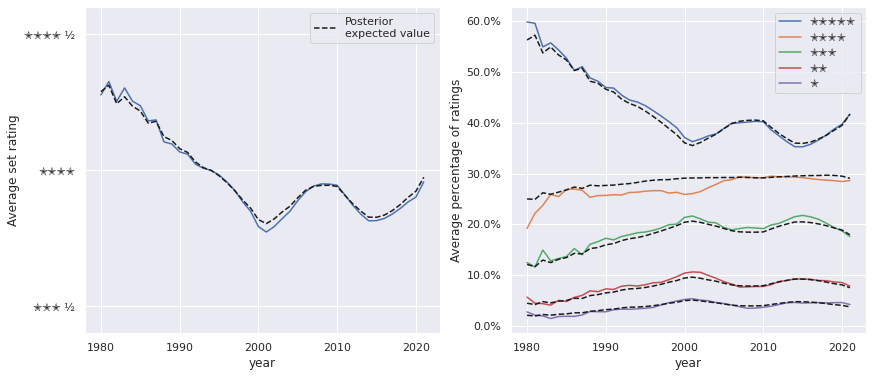We'll refine this model before we start interpreting set-level effects, but it is instructive to consider, even in this simple model, the effect of hierarchical shrinkage on sets with the fewest and the most reviews.

In :
def make_row_set_label(row):
return f"{row['Name']} ({row.name})"

def make_set_label(set_df):
return set_df.apply(make_row_set_label, axis=1)

In :
total_ratings_argsorted = ratings_ct.sum(axis=1).argsort()
fewest_total_ratings = total_ratings_argsorted[:10]
most_total_ratings = total_ratings_argsorted[-10:]

In :
fig, axes = plt.subplots(
ncols=2, sharex=True,
figsize=(1.75 * FIG_WIDTH, FIG_HEIGHT)
)

az.plot_forest(
trace, var_names=["ratings_obs_probs"],
transform=average_rating,
coords={"set": coords["set"][fewest_total_ratings]},
combined=True, ax=axes
);
axes.scatter(
rated_df["Average rating"]
.iloc[fewest_total_ratings]
.iloc[::-1]
.values,
axes.get_yticks(),
c='k', zorder=5,
label="Actual"
);

axes.set_xlabel("Average rating");
axes.set_yticklabels(make_set_label(
rated_df.iloc[fewest_total_ratings]
.iloc[::-1]
));

axes.legend();
axes.set_title("Sets with the fewest ratings");

az.plot_forest(
trace, var_names=["ratings_obs_probs"],
transform=average_rating,
coords={"set": coords["set"][most_total_ratings]},
combined=True, ax=axes
);
axes.scatter(
rated_df["Average rating"]
.iloc[most_total_ratings]
.iloc[::-1],
axes.get_yticks(),
c='k', zorder=5,
label="Actual"
);

axes.set_xlabel("Average rating");

axes.yaxis.tick_right();
axes.set_yticklabels(make_set_label(
rated_df.iloc[most_total_ratings]
.iloc[::-1]
));

axes.set_title("Sets with the most ratings");

fig.tight_layout();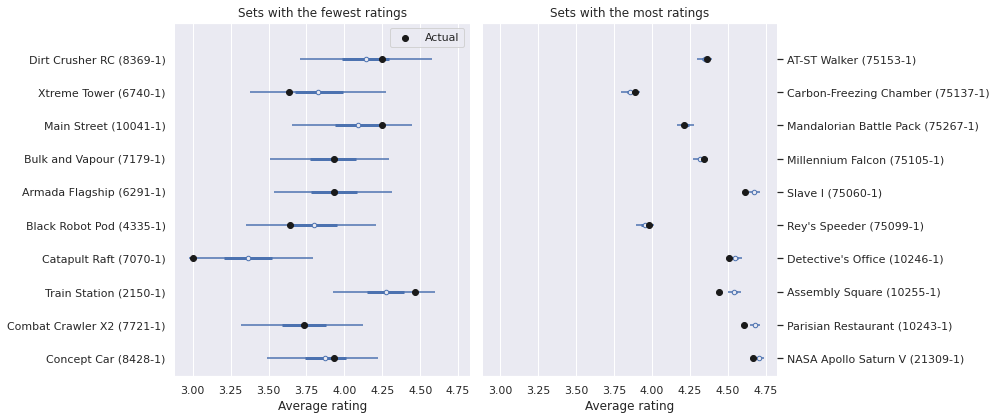There are two interesting elements in these plots. First is that the posterior credible intervals are much larger for the sets with the fewest ratings and for those with the most ratings. This makes perfect sense, as we have much more information about the sets on the right. The second is that the posterior expected ratings for the sets with the fewest ratings are significantly further from their true values than those for the sets with the most ratings. This is reflective of the fact that our hierarcical model shrinks the observed ratings towards the average rating for the year that set was released. This shrinkage applies more to sets with fewer reviews, resulting in the behavior show by the plot on the left.

#### Full model¶

The full model we will use to interpret set ratings takes into account not only the year in which the set was released but also its theme and subtheme (taxonomic categories of related sets) and its piece count and price.

This model includes year- and set-effects in the same way as the previous one.

In :
theme_id, theme_map = rated_df["Theme"].factorize(sort=True)

In :
sub_id, sub_map = (rated_df["Subtheme"]
.fillna("None")
.factorize(sort=True))
n_sub = sub_map.size

In :
coords["sub"] = sub_map
coords["theme"] = theme_map

In :
with pm.Model(coords=coords, rng_seeder=SEED) as full_model:
β_t_inc = pm.Normal("β_t_inc", 0., 0.1, dims="knot")
β_t = β_t_inc.cumsum()
f_t = pm.Deterministic("f_t", at.dot(t_dmat, β_t), dims="year")

β_set = noncentered_normal("β_set", dims="set", μ=0.)


As shown in our exploratory data analysis, there is a fairly linear relationship between log piece count and log RRP and a set's average rating.

In :
pieces_price_grid.fig

Out: